Latest Banking jobs   »

# Quantitative Aptitude Quiz For Bank of Baroda SO 2023 -26th May

Direction (1 – 10): In the given questions, two quantities are given, one as ‘Quantity I’ and another as ‘Quantity II’. You have to determine relationship between two quantities and choose the appropriate option:

Q1. The ratio between length and breadth of rectangle is 7: 4 and perimeter of rectangle is 88 cm.
Quantity I – Perimeter of square
Side of square is 6 cm less than length of rectangle.
Quantity II – Circumference of the circle
(a) Quantity I > Quantity II
(b) Quantity I < Quantity II
(c) Quantity I ≥ Quantity II
(d) Quantity I ≤ Quantity II
(e) Quantity I = Quantity II or no relation

Q2. Train ‘P’ running at the speed of 72 km/hr cross a man who running in opposite direction at the speed of 9 km/hr in 20 sec.
Quantity I – Length of platform
Length of train ‘Q’ is 70 meter more than that of train ‘P’ and is running at the speed of 90 km/hr and crosses a platform in 40 sec.
Quantity II – 520 m
(a) Quantity I > Quantity II
(b) Quantity I < Quantity II
(c) Quantity I ≥ Quantity II
(d) Quantity I ≤ Quantity II
(e) Quantity I = Quantity II or no relation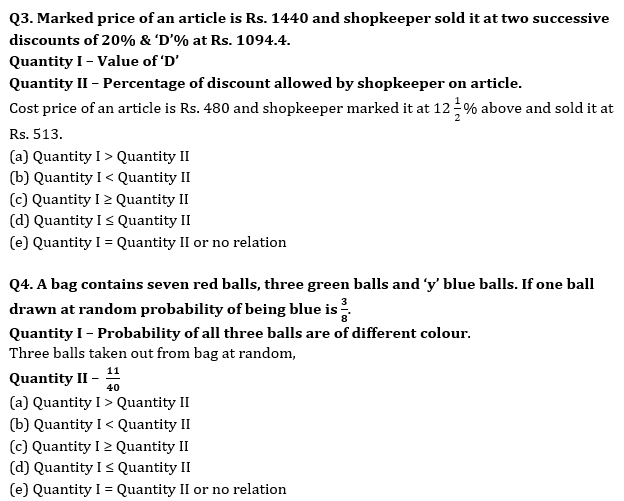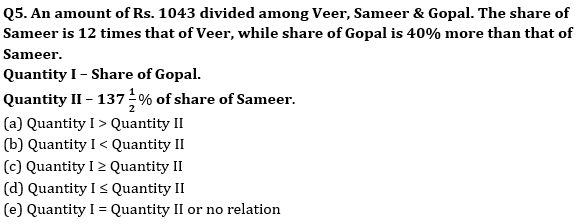Q6. (X – 4) men can complete a work in 5 days while (X + 5) men can complete the same work in 4 days. (Y – 15) women can complete a work in 6 days while (Y + 15) women can complete the same work in 3 days.
Quantity I: Value of ‘X’
Quantity II: Value of ‘Y’
(a) Quantity I > Quantity II
(b) Quantity I < Quantity II
(c) Quantity I ≥ Quantity II
(d) Quantity I ≤ Quantity II
(e) Quantity I = Quantity II or No relation

Q7. A and B starting a business together. B invested 60% more than that of A. Ratio of time period of investment of A and B is 8 : 9.
Quantity I: A’s share of profit out of total profit of Rs. 25,200.
Quantity II; B’s share of profit out of total profit of Rs. 14,000.
(a) Quantity I > Quantity II
(b) Quantity I < Quantity II
(c) Quantity I ≥ Quantity II
(d) Quantity I ≤ Quantity II
(e) Quantity I = Quantity II or No relation

Q8. Rs. ‘2X’ was invested half in scheme ‘A’ which offers 20% p.a. at S.I. while half in scheme ‘B’ which offer 10% p.a. at C.I. Total interest earned after 2 years is Rs. 4880.
Quantity I: Rs. (X + 2000)
Quantity II: 1.25X
(a) Quantity I > Quantity II
(b) Quantity I < Quantity II
(c) Quantity I ≥ Quantity II
(d) Quantity I ≤ Quantity II
(e) Quantity I = Quantity II or No relation

Q9. The probability of choosing two yellow balls out of eight balls in which three balls are red and five balls are yellow is ‘X’.
Quantity I: 8/27
Quantity II: X
(a) Quantity I > Quantity II
(b) Quantity I < Quantity II
(c) Quantity I ≥ Quantity II
(d) Quantity I ≤ Quantity II
(e) Quantity I = Quantity II or No relation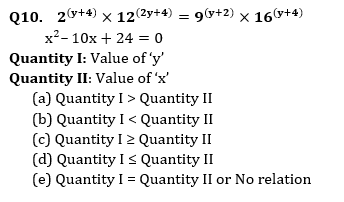Solutions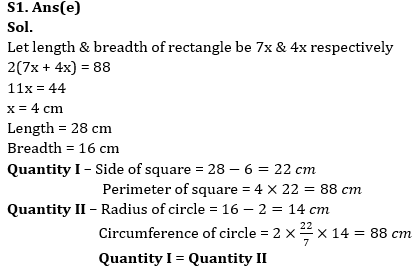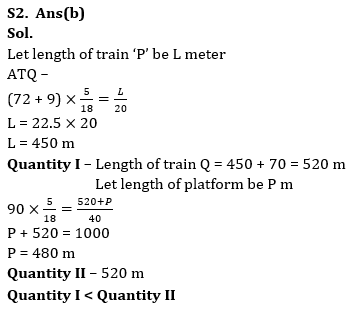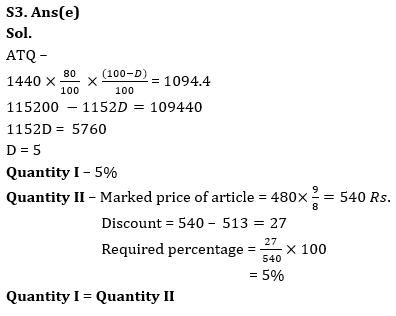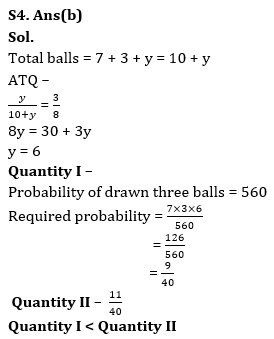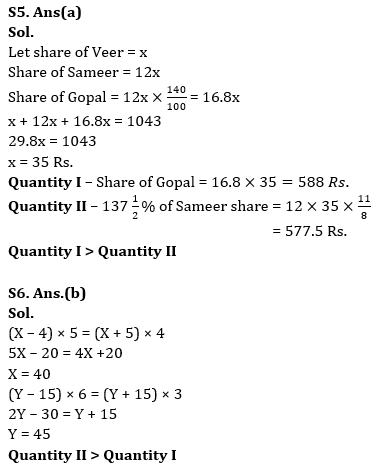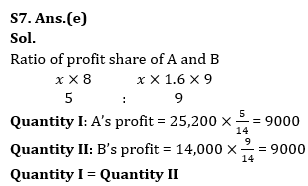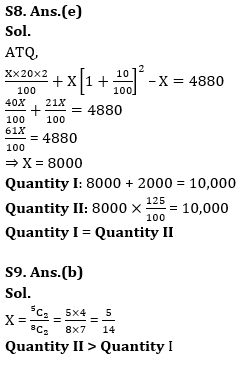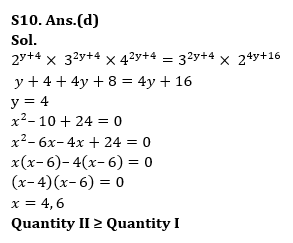## FAQs

### What is the selection process of Bank of Baroda AO 2023?

The selection process of Bank of Baroda AO consists of Online exam and Interview

#### Congratulations!Union Budget 2023-24: Free PDF